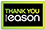# Polynomials, dynamics, and choice

PAPERBACK
Working out solutions to polynomial equations is a mathematical problem that dates from antiquity. Galois developed a theory in which the obstacle to solving a polynomial equation is an associated collection of symmetries. Obtaining a root requires "breaking" that symmetry. When the degree of an equation is at least five, Galois Theory established that there is no formula for the solutions like those found in lower degree cases. However, this negative result doesn't mean that the practice of equation-solving ends. In a recent breakthrough, Doyle and McMullen devised a solution to the fifth-degree equation that uses geometry, algebra, and dynamics to exploit icosahedral symmetry. Polynomials, Dynamics, and Choice: The Price We Pay for Symmetry is organized in two parts, the first of which develops an account of polynomial symmetry that relies on considerations of algebra and geometry. The second explores beyond polynomials to spaces consisting of choices ranging from mundane decisions to evolutionary algorithms that search for optimal outcomes. The two algorithms in Part I provide frameworks that capture structural issues that can arise in deliberative settings. While decision-making has been approached in mathematical terms, the novelty here is in the use of equation-solving algorithms to illuminate such problems. Features Treats the topic-familiar to many-of solving polynomial equations in a way that's dramatically different from what they saw in school Accessible to a general audience with limited mathematical background Abundant diagrams and graphics.
€60.19180 Reward Points
In stock online
Delivery in 2-3 working days

Any purchases for more than €10 are eligible for free delivery anywhere in the UK or Ireland!

Working out solutions to polynomial equations is a mathematical problem that dates from antiquity. Galois developed a theory in which the obstacle to solving a polynomial equation is an associated collection of symmetries. Obtaining a root requires "breaking" that symmetry. When the degree of an equation is at least five, Galois Theory established that there is no formula for the solutions like those found in lower degree cases. However, this negative result doesn't mean that the practice of equation-solving ends. In a recent breakthrough, Doyle and McMullen devised a solution to the fifth-degree equation that uses geometry, algebra, and dynamics to exploit icosahedral symmetry. Polynomials, Dynamics, and Choice: The Price We Pay for Symmetry is organized in two parts, the first of which develops an account of polynomial symmetry that relies on considerations of algebra and geometry. The second explores beyond polynomials to spaces consisting of choices ranging from mundane decisions to evolutionary algorithms that search for optimal outcomes. The two algorithms in Part I provide frameworks that capture structural issues that can arise in deliberative settings. While decision-making has been approached in mathematical terms, the novelty here is in the use of equation-solving algorithms to illuminate such problems. Features Treats the topic-familiar to many-of solving polynomial equations in a way that's dramatically different from what they saw in school Accessible to a general audience with limited mathematical background Abundant diagrams and graphics.
Quantity:
In stock online
Delivery in 2-3 working days180 Reward Points

Any purchases for more than €10 are eligible for free delivery anywhere in the UK or Ireland!

€60.19
In stock online
Delivery in 2-3 working days